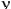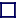### INERTIA and the SPEED OF LIGHT

A massive body (one which possesses a property called 'mass') can never travel as fast as the speed of light. Only bodies possessesing zero mass can travel at the speed of light, in fact, they cannot then travel at less than the speed of light, as light itself shows. [This is only true for light travelling in a vacuum, if travelling through some material medium, light does and will travel slower than c, the speed of light in a vacuum]. The photon has zero rest mass and only travels at the speed of light. Photons do have energy, and the energy of a photon is proportional to its frequency, E=h. [where h is Plancks constant, andis the frequency of the photon]. Einstein said that anything possessing energy, has mass, (E=mc2, but in the case of a photon, this is mass due to its energy; at zero frequency it has zero mass, but at zero frequency it is stationary and doesn't even exist. Higher frequency photons have higher energy.

Particles with non-zero rest mass (the mass they possess at zero velocity) must always travel slower than the speed of light. Mass is the reluctance of a particle to undergo any change in acceleration, which includes a change in direction. Massive bodies possess inertia: a massive particle will continue in a straight line unless acted upon by a force. To accelerate a massive body it must be supplied with extra energy, and this extra energy will increase its inertial mass [m=E/c2]. To accelerate it further it must be supplied with even more energy, but it needs ever more energy to accelerate it by a given amount because its inertial mass keeps increasing as its velocity increases! It can never be accelerated it up to the speed of light because it would need supplying with an infinite amount of energy and it would then possess infinite inertial mass. Mass is thought to be imposed upon particles by the Higgs Boson, which also imposes a mass upon itself.

Mach told us that force due to gravity and force due to acceleration are indistinguishable. If a person was in a capsule, unable to see outside, he would be un-able to tell if either the capsule was stationary on Earth, or it was being accelerated in a space rocket at an acceleration of 1g (which applies the same force as Gravity does on Earth), the force on his body would be identical. If, on the other hand, he felt weightless inside the capsule, he would be then un-able to tell if the capsule was in free-fall plummeting towards Earth, or the rocket had stopped accelerating (but might still be travelling at a velocity of 20,000 km/second). That is Mach's Principle: the equivalence of force and acceleration.

But it doesn't end there. Mass exerts a gravitational force itself. It has a gravitational field around it. Two massive particles alone in space will attract each other and accelerate each other towards themselves. But a gravitational force can also be regarded as a warp in space. A massive body pulls space itself a little towards itself, the more massive the body, the more it warps the space around itself, until in the extreme case it tears itself away from the rest of space and becomes a Black Hole. This is the true nature of mass, gravity and acceleration. Around a massive body, space itself is non-linear.